# Test: Inequalities in a Triangle

## 15 Questions MCQ Test Mathematics (Maths) Class 9 | Test: Inequalities in a Triangle

Description
Attempt Test: Inequalities in a Triangle | 15 questions in 15 minutes | Mock test for Class 9 preparation | Free important questions MCQ to study Mathematics (Maths) Class 9 for Class 9 Exam | Download free PDF with solutions
QUESTION: 1

Solution:
QUESTION: 2

Solution:
QUESTION: 3

### Which of the following does not represent the sides of a triangle?

Solution:
QUESTION: 4

In figure, which of the following statement is true?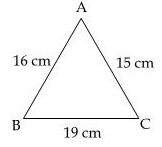Solution: Since, angle opposite to the smallest side is the smallest angle,<b (opposite to smallest side AC = 15cm) is the smallest angle.
QUESTION: 5

In triangle ABC, ∠B = 35°, ∠C = 65° and the bisector of ∠BAC meets BC in P, choose the correct order of inequality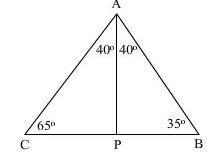Solution:
QUESTION: 6

If length of the largest side of a triangle is 12 cm then other two sides of triangle can be:​

Solution:
QUESTION: 7

Two sides of triangle are of lengths 5 cm and 1.5 cm. The length of the third side of the triangle cannot be​

Solution:
QUESTION: 8

In a triangle ABC this statement will always be true.

Solution:
QUESTION: 9

In ΔPQR, S is any point on the side QR, then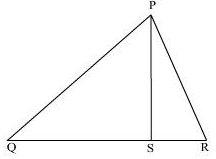Solution:
QUESTION: 10

In ΔABC, ∠A = 40° and ∠B = 60°. The longest side is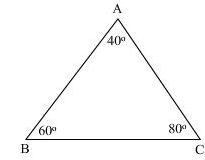Solution:
QUESTION: 11

The perimeter of a triangle is…. the sum of its medians.

Solution:
QUESTION: 12

It is not possible to construct a triangle when its sides are:​

Solution:
QUESTION: 13

Which of the following is incorrect ?

Solution:
QUESTION: 14

The figure shows that O is the centre of the circle and XOY is a diameter. If XZ is any other chord of the circle, then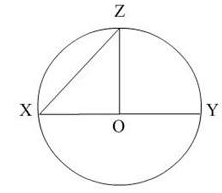Solution:
QUESTION: 15

In fig., which of the following statement is true?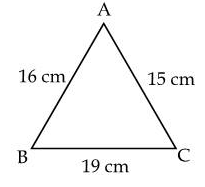Solution:Use Code STAYHOME200 and get INR 200 additional OFF Use Coupon Code